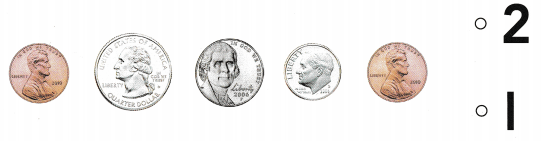# Texas Go Math Kindergarten Lesson 15.4 Answer Key Quarter

Refer to our Texas Go Math Kindergarten Answer Key Pdf to score good marks in the exams. Test yourself by practicing the problems from Texas Go Math Kindergarten Lesson 15.4 Answer Key Quarter.

## Texas Go Math Kindergarten Lesson 15.4 Answer Key Quarter

Essential Question
How do you identify, describe, and name quarters?

Explore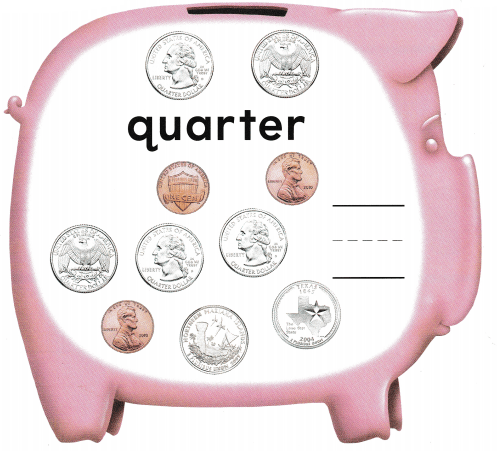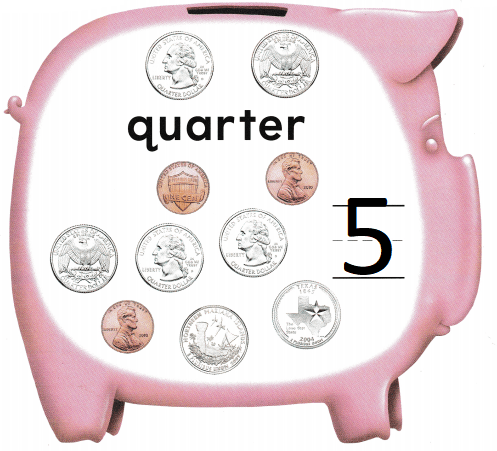Explanation:
A quarter is worth 25 cents.
There are 5 quarters in the group
and there are 3 pennies.

Directions
Place a quarter to match each one shown at the top of the page. Tell what is alike about the quarters. Tell what is different about the quarters. Circle the quarters in the set at the bottom of the page. Write the number of quarters.

Share and Show

Question 1.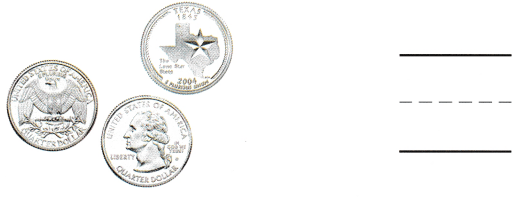Explanation:
A quarter is worth 25 cents.
There are 3 quarters in the group

Question 2.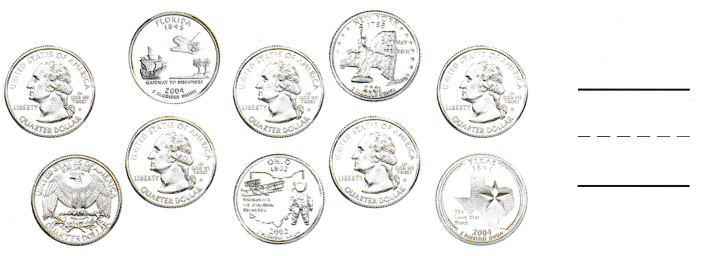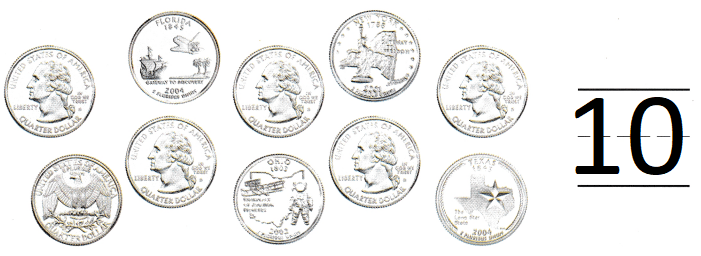Explanation:
A quarter is worth 25 cents.
There are 10 quarters in the group

Question 3.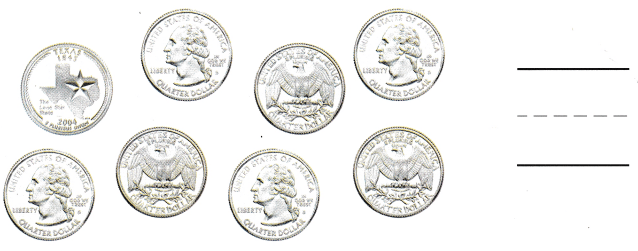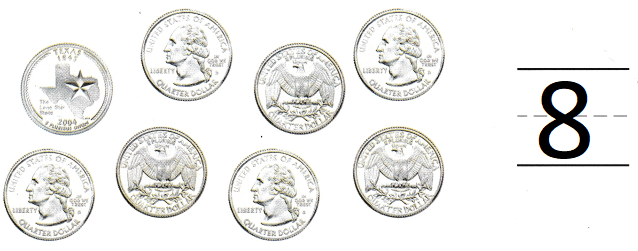Explanation:
A quarter is worth 25 cents.
There are 8 quarters in the group

Directions
1-3. Count the quarters. Write the number that shows how many quarters.

Question 4.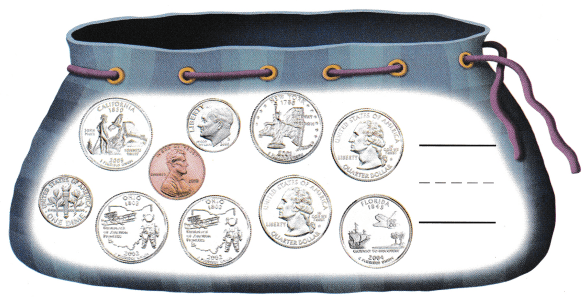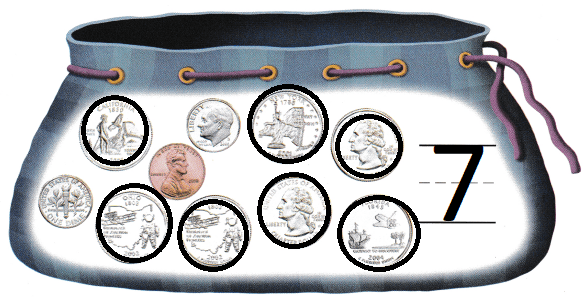Explanation:
A quarter is worth 25 cents.
There are 7 quarters in the bag

Question 5.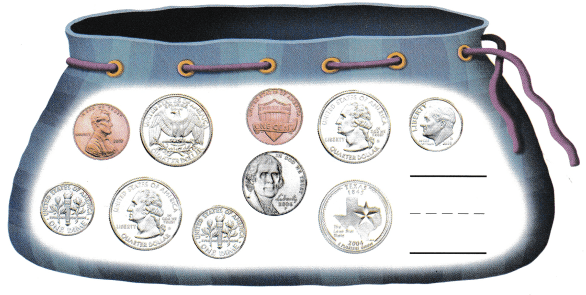Explanation:
A quarter is worth 25 cents.
There are 4 quarters in the bag

Directions
4-5. Circle the quarters. Count and write the number of quarters.

Home Activity

• Show your child a quarter. Hove him or her tell you the name of the coin and describe both sides.

Problem Solving

Question 6.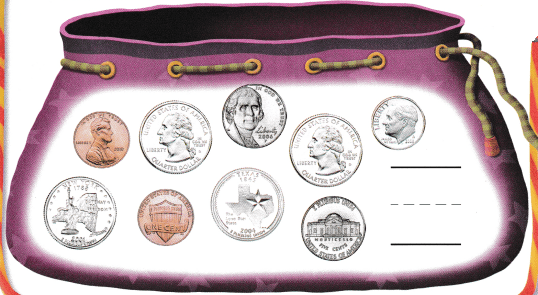Explanation:
Julia needs four quarters to buy a book
There are 4 quarters in the bag
so, Julia has sufficient money to buy a book

Question 7.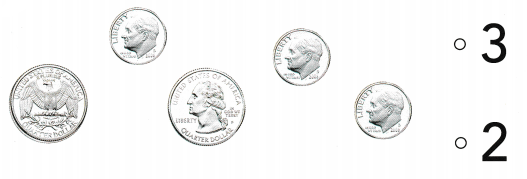Explanation:
A quarter is worth 25 cents.
There are 2 quarters in the group

Directions
6. Julia needs four quarters to buy a book. Count the quarters to see if she has enough. Circle the quarters if she has enough. Write the number of quarters. 7. Choose the correct answer How many quarters are in this group?

### Texas Go Math Kindergarten Lesson 15.4 Homework and Practice

Question 1.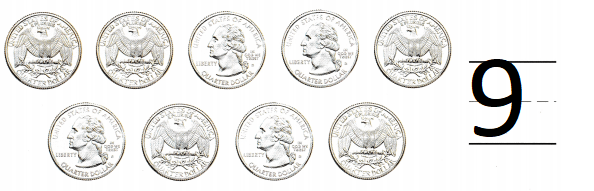Explanation:
A quarter is worth 25 cents.
There are 9 quarters

Question 2.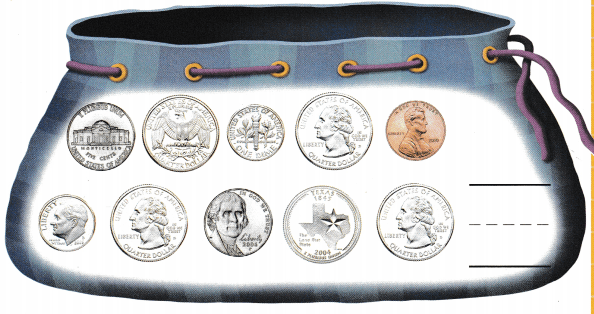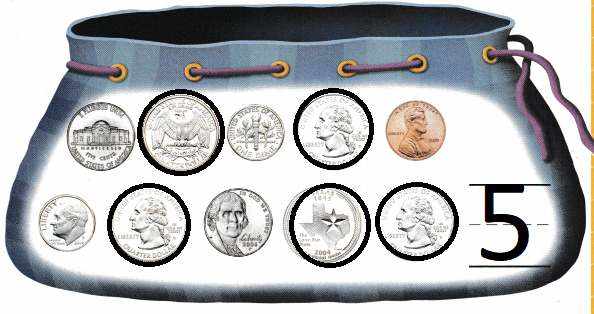Explanation:
A quarter is worth 25 cents.
In the bag there are 5 quarters

Directions
1. Count the quarters. Write the number that shows how many quarters. 2. Circle the quarters. Count and write the number of quarters.

Texas Test Prep

Lesson Check

Question 3.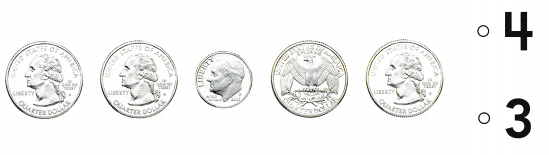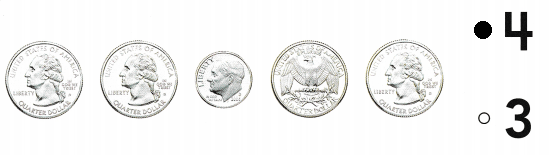Explanation:
A quarter is worth 25 cents.
There are 4 quarters

Question 4.Explanation:
A quarter is worth 25 cents.
There are 3 quarters

Question 5.